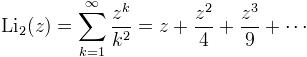Computes the dilogarathm function, also called Spence's function.

Namespace:  Meta.Numerics.Functions
Assembly:  Meta.Numerics (in Meta.Numerics.dll) Version: 4.1.4Syntax
```public static double DiLog(
double x
)```

#### Parameters

x
Type: SystemDouble
The argument, which must be less than or equal to unity.

#### Return Value

Type: Double
The value Li2(x).Remarks

The dilogarithm can be defined by an infinite sum.The function gets is name from the similarity of this series to the expansion of ln(1-x), the difference being that the integer in the denominator is raised to the second power.

Li2(x) is real for -∞ < x ≤ 1; for values outside this range, use the complex version DiLog(Complex).See Also